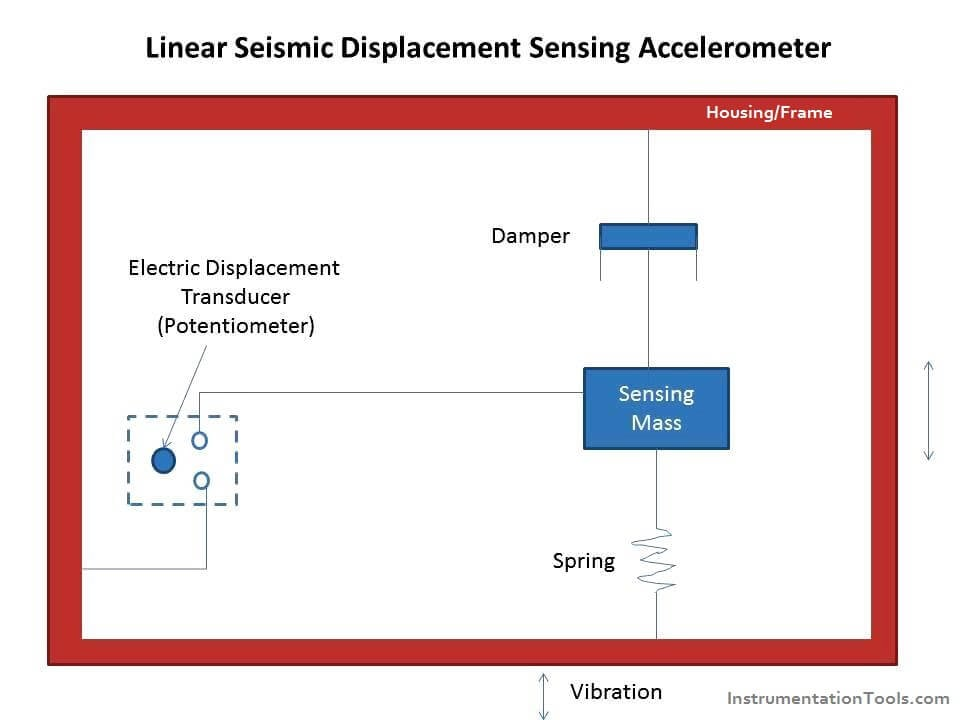# Seismic Displacement Sensing Accelerometer

When a spring – mass – damper system is subjected to acceleration, the mass is displaced, and this displacement of the mass is proportional to the acceleration. Hence a measure of displacement of the mass becomes a measure of acceleration.The main parts of a seismic accelerometer are as follows:

1. A seismic mass is suspended from the housing of the accelerometer through a spring.
2. A damper is connected between the seismic mass and the housing of the accelerometer.
3. The seismic mass is connected to an electric displacement transducer.

Note: there are two types of seismic – displacement sensing accelerometers namely.

1. Linear seisimic accelerometer.
2. Rotational Seismic Accelerometer.

Both of the above mentioned seismic accelerometers work on the same principle and are shown in diagram.#### Operation

1. The accelerometer is fitted on to the structure whose acceleration is to be measured.
2. Due the acceleration, the seismic mass experience a displacement and this displacement of the mass is proportional to the acceleration.
3. As the mass is connected to an electric displacement transducer, the output of the transducer depends on the extent – to which the mass is displaced.
4. Hence the output of the transducer is calibrated to give a direct indication of the acceleration characteristics of the structure.